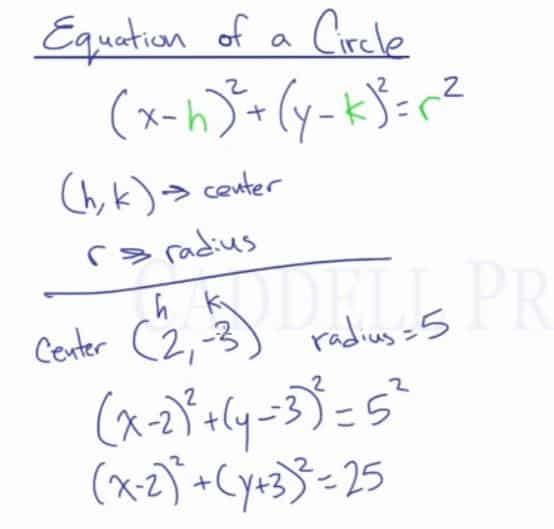In this video lesson, you will learn the equation of a circle and how to identify the center of the circle and its radius if given the equation or a circle.

Equation of a Circle$(x-h)^2+(y-k)^2=r^2$
where (h,k) is the coordinate point for the center of the circle and r is the radius.

For example:
If the center is (2,-3) and the radius is 5, then the equation of the circle is:$(x-2)^2+(y+3)^2=5^2$$(x-2)^2+(y+3)^2=25$Let’s use the equation of the circle to find the coordinate points:

For example:
If the equation of the circle is$(x+6)^2+(y-5)^2=36$

Since we know that the x-value inside the parenthesis is always the reverse operation of the coordinate point, the center would be$(-6,5)$.

The radius is always the square root of the value on the other side.$36$$\sqrt{36}$$6$

Center:$(-6,5)$
Radius:$6$

## Video-Lesson Transcript

Equation of a circle.

The general equation of a circle is$(x - h)^{2} + (y - k)^{2} = r^2$

where$(h, k)$ are the coordinates for the center of the circle and$r$ is the radius.

For example, if the center of a circle is$(2, -3)$ and the radius is$5$.

The equation of a circle is$(x - h)^{2} + (y - k)^{2} = r^2$$(x - 2)^{2} + (y - -3)^{2} = 5^2$$(x - 2)^{2} + (y + 3)^{2} = 25$

The last part is always the radius squared.

Something to notice is that whatever signs$h$ and$k$ have, it’s going to be the opposite of what’s in the formula.

Now, let’s look at another example.$(x + 6)^2 + (y - 5)^2 = 36$

The center of the circle is$(-6, +5)$. The signs are opposite on what is in the formula.

The radius is$6$ because$\sqrt{36} = 6$.

Let’s look at another one.$(x - 4)^2 + (y + 9)^2 = 13$

So, the center is$(4, -9)$.

The radius is$\sqrt{13}$.

Keep in mind that whatever sign you have in the formula is the opposite sign for the center of the circle.

And the last part is always radius squared. So, the radius is the square root of the last number.